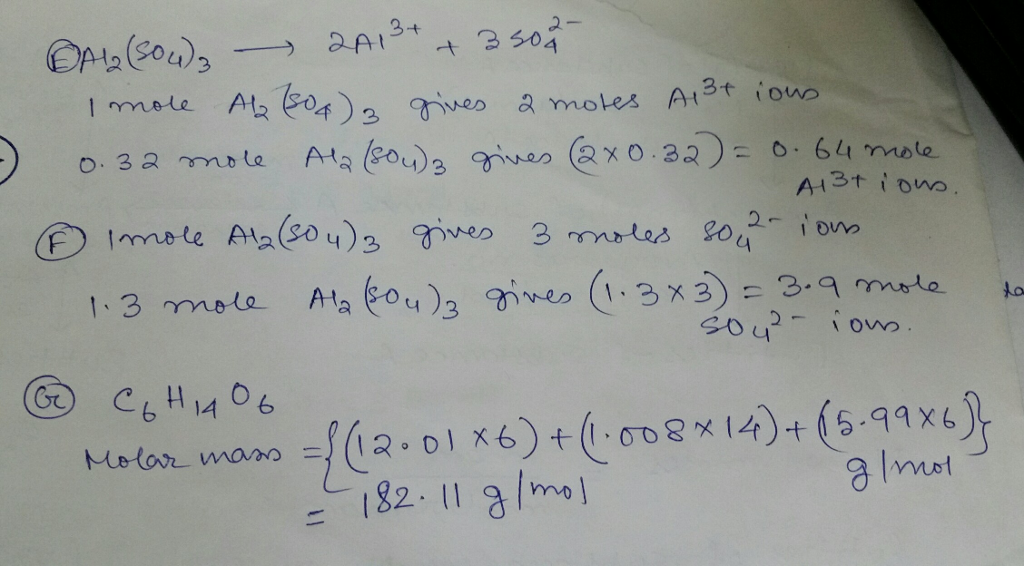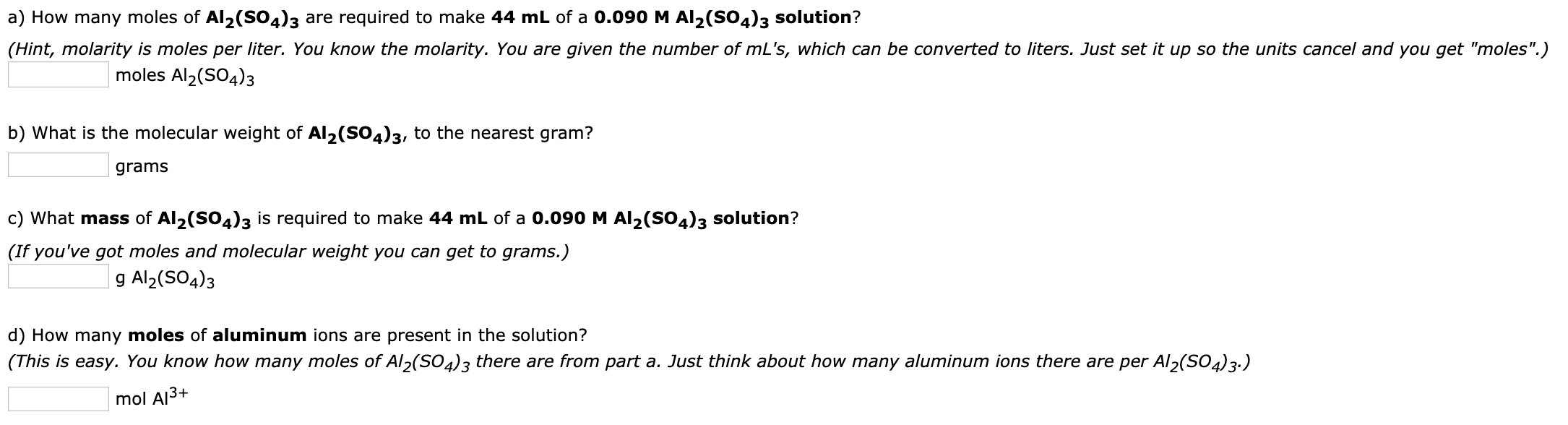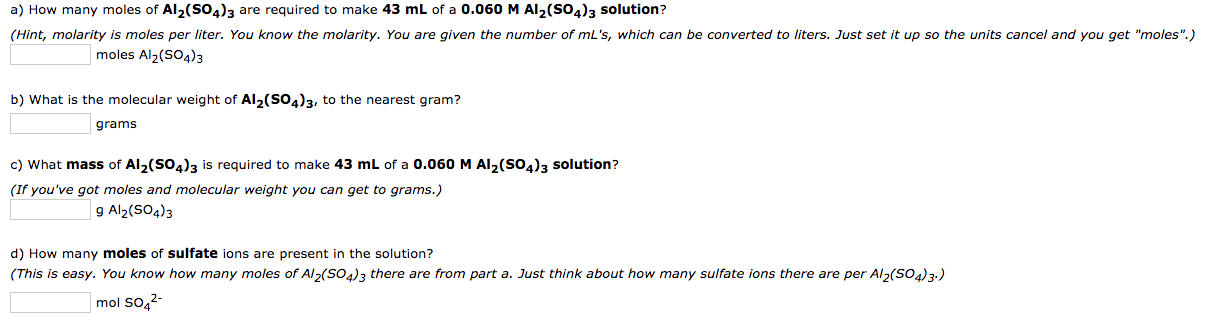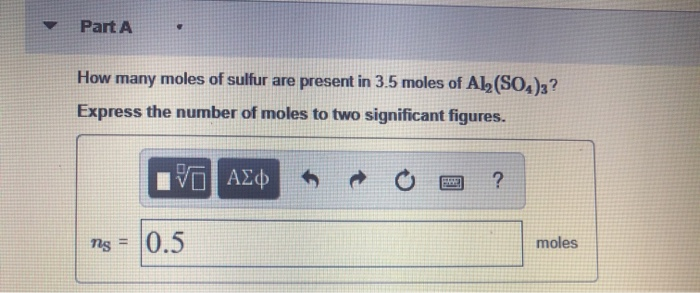Question

# E: How many moles of aluminum ions are present in 0.32 moles of Al2(SO4)3? F: How...

E: How many moles of aluminum ions are present in 0.32 moles of Al2(SO4)3?

F: How many moles of sulfate ions (SO42?) are present in 1.3 moles of Al2(SO4)3?

G: Calculate molar mass C6H14O6 (sorbitol) Express your answer to two decimal places and include the appropriate units.

H: The sedative Demerol hydrochloride has the formula C15H22ClNO2. How many grams are in 0.025 moles of Demerol hydrochloride?

I: How many moles of H2 are needed to react with 0.55 mol of N2?

J: How many moles of N2 reacted if 0.85 mol of NH3 is produced?

K: How many moles of NH3 are produced when 1.7 mol of H2 reacts?

L: Calculate the mass of water produced when 2.07 g of butane reacts with excess oxygen.

M: Calculate the mass of butane needed to produce 46.2 g of carbon dioxide.

N: If you have 3.17 g of H2, how many grams of NH3 can be produced?

O: How many grams of H2 are needed to react with 2.90 g of N2?

P:How many grams of NH3 can be produced from 18.0 g of H2?

Q: How many grams of glucose are produced from 23.0 g of CO2?

R: How much heat, in kilojoules, is needed to produce 23.0 g of C6H12O6?#### Earn Coins

Coins can be redeemed for fabulous gifts.

Similar Homework Help Questions
• ### a) How many moles of Al2(SO4)3 are required to make 44 mL of a 0.090 M Al2(SO4)3 solution? (Hint, molarity is moles per...a) How many moles of Al2(SO4)3 are required to make 44 mL of a 0.090 M Al2(SO4)3 solution? (Hint, molarity is moles per liter. You know the molarity. You are given the number of mL's, which can be converted to liters. Just set it up so the units cancel and you get "moles".) moles Al2(SO4)3 b) What is the molecular weight of Al2(SO4)3, to the nearest gram? grams c) What mass of Al2(SO4)3 is required to make 44 mL of...

• ### a) How many moles of Al2(SO4)3 are required to make 55 mL of a 0.050 M...

a) How many moles of Al2(SO4)3 are required to make 55 mL of a 0.050 M Al2(SO4)3 solution? (Hint, molarity is moles per liter. You know the molarity. You are given the number of mL's, which can be converted to liters. Just set it up so the units cancel and you get "moles".) moles Al2(SO4)3 b) What is the molecular weight of Al2(SO4)3, to the nearest gram? grams c) What mass of Al2(SO4)3 is required to make 55 mL of...

• ### How many moles of aluminum ions are present in 0.40 mole of Al2(SO4)3

How many moles of aluminum ions are present in 0.40 mole of Al2(SO4)3

• ### a) How many moles of Al2(SO4)3 are required to make 43 mL of a 0.060 M...a) How many moles of Al2(SO4)3 are required to make 43 mL of a 0.060 M Al2(So4)3 solution? (Hint, molarity is moles per liter. You know the molarity. You are given the number of mL's, which can be converted to liters. Just set it up so the units cancel and you get "moles".) moles Al2(SO4)3 b) What is the molecular weight of Al2(S04)3, to the nearest gram? grams c) What mass of Al2(SO4)3 is required to make 43 mL of...

• ### a) How many mol of Al2(SO4)3 are required to make 40 mL of a  0.050 M Al2(SO4)3...

a) How many mol of Al2(SO4)3 are required to make 40 mL of a  0.050 M Al2(SO4)3 solution? (Hint, molarity is moles per liter. You know the molarity. You are given the number of mL's, which can be converted to liters. Just set it up so the units cancel and you get "moles".) moles Al2(SO4)3 b) What is the molecular weight of Al2(SO4)3, to the nearest gram? c) What mass of Al2(SO4)3 is required to make 40 mL of a  0.050 M...

• ### How many moles of sulfate ions are present in 2.4 moles of Al2(SO4)3?

How many moles of sulfate ions are present in 2.4 moles of Al2(SO4)3?

• ### Part A How many moles of sulfur are present in 3.5 moles of Al2(SO4)3? Express the...Part A How many moles of sulfur are present in 3.5 moles of Al2(SO4)3? Express the number of moles to two significant figures. V AX“ o ? 0.5 moles Part B How many moles of aluminum ions are present in 0.36 moles of Al(SO4)3? Express the number of moles to two significant figures. VO AEM O O ? TAI = 0.6 mol Submit Previous Answers Request Answer Part How many moles of sulfate ions (S0,2 - ) are present in...

• ### 3H2(g)+N2(g)→2NH3(g) a. How many moles of NH3 can be produced from 13.5 mol of H2 and...

3H2(g)+N2(g)→2NH3(g) a. How many moles of NH3 can be produced from 13.5 mol of H2 and excess N2? b. How many grams of NH3 can be produced from 4.90 mol of N2 and excess H2. c. How many grams of H2 are needed to produce 12.74 g of NH3? d. How many molecules (not moles) of NH3 are produced from 1.05×10−4 g of H2?

• ### 2Al+3H2SO4⟶3H2+Al2(SO4)3 how many moles of hydrogen will be produced from 17.6 g of aluminum?

2Al+3H2SO4⟶3H2+Al2(SO4)3 how many moles of hydrogen will be produced from 17.6 g of aluminum?

• ### 3 H2 + N2 → 2 NH3 1. If you have 3.22 moles of H2, how...

3 H2 + N2 → 2 NH3 1. If you have 3.22 moles of H2, how many moles of NH3 can you make? 2. If you have 1.19 moles of H2, how many moles of NH3 can you make? 3. If you have 15.22 moles of N2, how many moles of NH3 can you make? 4. How many moles of H2 do you need to react with 5.55 moles of N2? 5. How many moles of NH3 can be made...